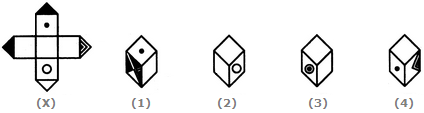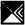# Non Verbal Reasoning - Cubes and Dice - Discussion

### Discussion :: Cubes and Dice - Section 1 (Q.No.12)

The sheet of paper shown in the figure (X) given on the left hand side, in each problem, is folded to form a box. Choose from amongst the alternatives (1), (2), (3) and (4), the boxes that are similar to the box that will be formed.

12.

Choose the box that is similar to the box formed from the given sheet of paper (X).[A]. 1 and 2 only [B]. 1, 2 and 3 only [C]. 1 and 3 only [D]. 1, 2, 3 and 4

Answer: Option A

Explanation:

The fig. (X) is similar to the Form VI. So, when a cube is formed by folding the sheet shown in fig. (X), thenis one of the faces of the cube and this face lies opposite to a blank face. Also, a face bearing a circle lies opposite to one bearing a dot. Clearly, this cube does not have faces as shown in the cubes in figures (3) and (4). Hence, only the cubes shown in figures (1) and (2) can be formed.

 Vish said: (Apr 4, 2018) Is there any simple & a quick technique to resolve this? Please explain the answer.

#### Post your comments here:

Name *:

Email   : (optional)

» Your comments will be displayed only after manual approval.

#### Current Affairs 2021

Interview Questions and Answers